﻿ D-Wave (annealing) is really a quantum computer ?

# D-Wave is a "real" quantum computer ?

## D-Wave system is a "real" quantum computer ?

(Fig.1) D-Wave machine based on quantum tunneling is real ?In optimization ( salesman ) problems, he needs to visit every city and try all possibilities to know the shortest path. But it takes almost infinite time, so impossible in some cases.

It is said that D-Wave machine with 512 qubits can solve this problem using quantum tunnelling and annealing, as shown on this site and this site.

Quantum annealing is a method for finding the global minimum from many candidate states using tunneling.
This D-Wave computer is different from traditional quantum computer (= impractical ), because it does NOT rely on entanglement.

## D-Wave computer showed NO quantum speed-up.

(Fig.2) D-Wave is NOT faster than classical computer.As shown in this news and this news, independent research group found NO quantum speed-up and advantage over conventional classical computer in the recent experiments using D-Wave.

D-Wave is the Only commercial adiabatic quantum computer, so this result showed that there is NO evidence of quantum computer in the real world, now.

Though D-Wave system is a private company, the paper about its machine is published in journal Science.
So I decided to write about D-Wave quantum computer.

## "Tunneling" in D-Wave computer is really "quantum" ?

(Fig.3) Quantum tunneling in D-Wave computer really uses quantum mechanics ?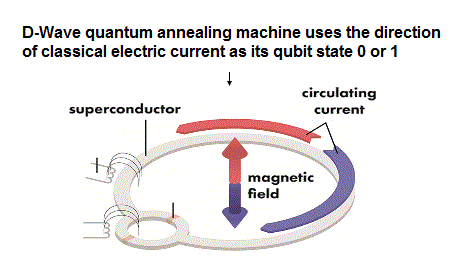The reason why D-Wave is called "quantum computer" is quantum tunneling.
It is said that quantum tunneling cannot be explained by classical mechanics.

We want to know whether D-Wave really uses purely quantum mechanical phenomena as tunneling.
By the way, what does "Quantum" phenomenon really mean ?

On this page, we show that definition of quantum barrier can be replaced by classical (= realistic ) mechanics in the tunneling in D-Wave.

## What causes sinusoidal curve of Josephson current ?

(Fig.4) What is the origin of Josephson corrent I(t) ?As shown on this site and this site (p.4), the electric current through Josephson junction becomes periodic.
Approximately, this periodicity is related to magnetic flux (= Φ ) through current loop.

## Magnetic flux quantum (= Φ0 ) is related to de Broglie wavelength.

(Fig.5) Integer number of de Broglie wavelength (= magnetic flux quantum ).It is known that magnetic flux through superconducting loop tends to be an integer times flux quantum ( n × Φ0 ). See also this section.

Though quantum mechanics avoids clarifying this origin of flux quantum, de Broglie relation clearly plays an important role, as shown on this site and this site (p.23).

This sinusoidal curve based on de Broglie waves is the origin of double well potential in the quantum tunneling of D-Wave computer.

## Induced voltage is given by the time derivative of magnetic flux.

(Fig.6) Induced voltage V by Faraday's law.According to Faraday's ( Lenz's ) law, the voltage is induced along the circuit, when the magnetic flux (= Φ ) changes with time.

This voltage produces a current whose magnetic field opposes the change which produces it.

## Change of electric energy = voltage V × current.

(Fig.7) The time rate of change of electric potential = V × I.The time rate of change of electric (= Coulomb ) energy is given by the voltage V × current I
( The current I is the amount of charges passing per second. )

The important point is that they use special Josephson current of Fig.4 in calculating Coulomb potential.
As I said, the origin of the sinusoidal curve of this current I is NOT electric force, but de Broglie relation.

So we have to consider NOT only Coulomb energy but also other (= de Broglie ) energy to get correct total potential (= barrier ? ) energy.

## Josephson energy (= EJ ) is self-contradictory.

(Fig.8) They neglect de Broglie effect to get Josephson energy.As shown on this site and this site, they rely ONLY on Coulomb relation to obtain total Josephson energy.
Total energy (= EJ ) is given by integrating I V (= current × voltage ) with respect to the time t.

This is clearly inconsistent.
Because the sinusoidal curve of the Josephson current I ( depending on the magnetic flux ) is caused by other effects than ordinary Coulomb potential

These other effects are clearly due to de Broglie wave nature.

(Fig.9) Ordinary magnetic energy in solenoidThe current loop has the property of solenoid with inductance L.
So as shown on this site (p.3) and this site, the energy stored in the magnetic field is given by Fig.9.

The magnetic flux (= Φ ) through this loop is equal to "LI" (= inductance × current ).

## Total energy of superconducting qubit ?

(Fig.10) Total energy = Josephson + magnetic energies ?Summing the effects of Fig.8 (= Josephson energy ) and Fig.9 (= ordinary magnetic energy ), total energy becomes like Fig.10.

ΦX is the magnetic flux from the external source, which is used for manipulating the total flux.
When this ΦX is a half of magnetic flux quantum (= 1/2Φ0 ),

(Fig.11)   Total energy ?The part of cosine (= Josephson energy ) causes, what we call, double-well potential in the current loop, as shown in Fig.12 and this site (p.9).

(Fig.12) What is the origin of potential barrier ?As shown in Fig.11 and Fig.12, when the phase θ is equal to 0 and 2π ( Φ = 0 and Φ0 ), the total potential becomes the lowest.

The difference of magnetic flux is generated by clockwise and counterclockwise Josephson current.

So they insist these two opposite currents are in superposition state ( when ΦX = 1/2Φ0 ), and can be used as qubit of quantum computer.

(Fig.12') Counterclockwise current = spin up ?   clockwise = spin down ? ← superposition ?Of course, many-world superposition is completely unrealistic.
These states just show the same probability in the directions of electric current I.

So, this phenomenon indicates some unknown forces are working, which tries to become an integer number of magnetic flux quantum (= n × Φ0 ).

## Classical Faraday law does NOT make double-well potential.

(Fig.13) Faraday law tries to keep the origical magnetic flux.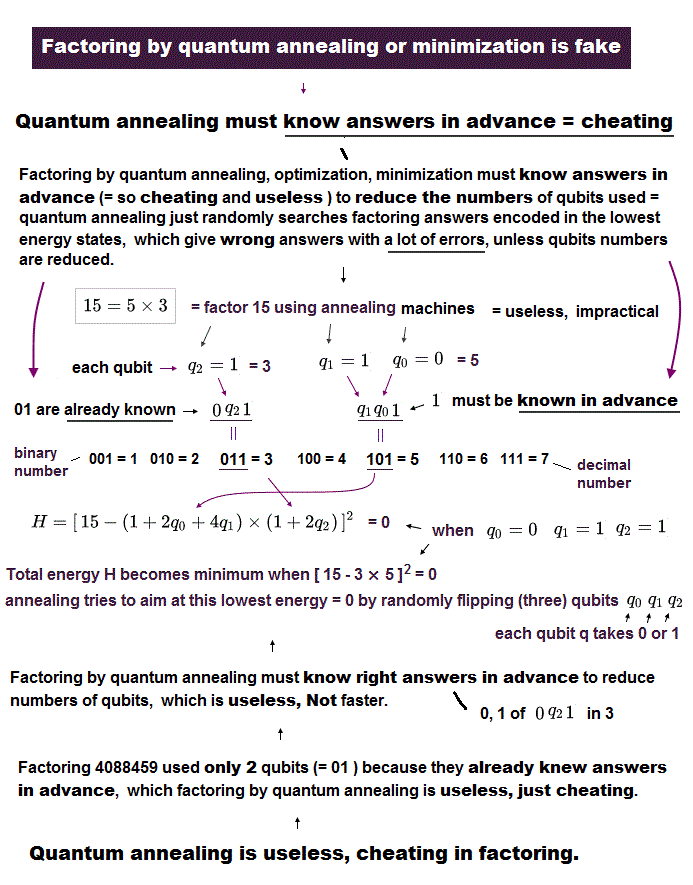Classical Faraday law tries to keep the original magnetic flux constant.
So when the magnetic flux decreases from the original 1/2Φ0, the induced voltage causes the current, which increases the magnetic flux.

This means the potential is lower in the original 1/2Φ0 in the ordinary electromagnetism.

## Magnetic flux increases → induced voltage causes "opposite" current.

(Fig.14) Faraday law tries to obstruct the increase in magnetic flux.When the magnetic flux increases from the original 1/2Φ0, the induced voltage generates the opposite current, which decreases the magnetic flux.

As a result, the potential energy in the original 1/2Φ0 is the lowest, if ONLY classical electromagnetism is related, so double-well potential is NOT related to the ordinary electric force.

## Classical electromagnetism has Nothing to do with double-well potentials.

(Fig.15)   Double-well potential (= barrier ) is caused by de Broglie effect.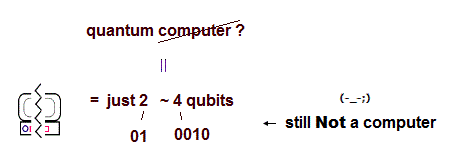D-Wave quantum computer claims the quantum tunneling can go through the barrier of double-well potentials in superconducting circuit, as shown on this paper ( Nature ).

But as shown in Fig.8, the calculation of this potential barrier is based ONLY on Coulomb relation (= current × V ), though this double-well is caused by de Broglie effect.

## de Broglie effect is the origin of double-well potential.

(Fig.16) An integer number of de Broglie wavelength is the origin of doube-well.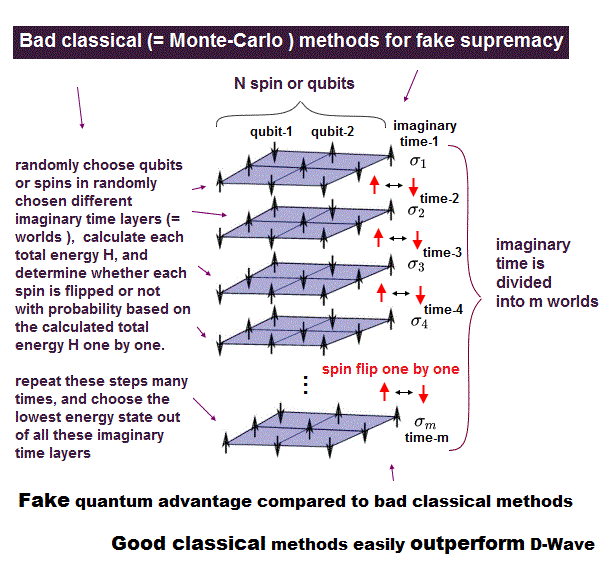As I said in Fig.5 and this section, the Josephson ( superconducting ) current tends to generate an integer number of magnetic flux quantum (= Φ0 ).

So when the magneic flux increases from the original 1/2Φ0, it tries to increase more to generate 1 × Φ0.
Of course, this driving force is NOT due to the original Faraday law.

Because the original Faraday law tries to suppress the increase of the current.
As a result, at this point, we have to consider other potentials than the ordinary Coulomb barrier.

## Ordinary electromagnetic energy cannot give double-well potentials.

(Fig.17)As shown in Fig.17, if ONLY the ordinary electromagnetic relation is related in superconducting circuit, the double-well potential cannot form.

Because the magnetic energy is proportional to the square of magnetic flux (= Φ2 ).

## de Broglie effect is the main cause of double-well potential.

(Fig.18)   De Broglie effect is the main reason of double-well.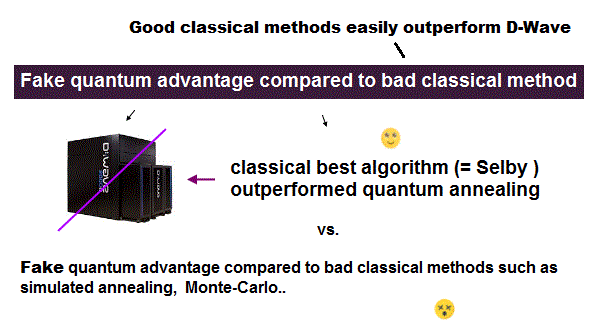In Fig.18, when the magnetic flux decreases from the original 1/2Φ0, it tries to decrease more to be the state of 0 × Φ0 (= integer × magnetic flux quantum ).

As I said, this effect cannot be explained ONLY by usual electromagnetic force ( potential ).

## Calculation of Josephson energy (= EJ ) is self-contradictory.

(Fig.19)   We have to consider other effects than Coulomb potential (= barrier ).But they depend ONLY on the usual electromagnetic relation (= current I × voltage V ) in calculating the sinusoidal curve of Josephson energy (= EJcosθ ).

In superconducting qubits, they claim the validity of quantum tunneling, based on the equation of Fig.19.
But Fig.19 is NOT complete, because it does NOT contain other important effects such as de Broglie relation.

Also in this paper ( Science ), they just artificially define the meaning of "quantum" and "classical" in magnetic susceptibility. So true "classical" (= in the realistic sense ) has NOT been disproved yet.

## D-Wave quantum computer.

### [ Opposite currents = many-world like superposition !? ]

(Fig.20) Counterclockwise current = spin up ?   clockwise = spin down ?   ← qubits ?From here, we explain about the original paper (= Nature ) and this paper of D-Wave quantum computer.

For example, when the magnetic flux through the current loop is 1/2Φ0, two different superpositions states (= 1 × Φ0 and 0 × Φ0 ) exist at the same time according to the current theory.

These contains two kinds of counterclockwise and clockwise currents, which they named spin-up and spn-down, though they are NOT real spin. These two states are used as qubits.

## Quantum tunneling in D-Wave computer ?

(Fig.21) Quantum tunneling or classical "thermal" effects.They can control two kinds of external magnetic fluxes to change the height of barrier and depth of two-wells.
Annealing (= tunneling ) is performed by gradually raising the energy barrier between two states.

In classical thermal annealing, when the barrier becomes much larger than kBT (= Boltzmann factor ) at some time, its thermal excitation over the barrier ceases, and tunneling stops, they insist.

(Fig.22) Barrier becomes higher with time → When (= tfreeze ) tunneling stops ?Also in quantum annealing, tunneling between two states will cease, at some time (= tfreeze ), when the barrier becomes high enough.

So if the potential (= height ) of the right-well becomes lower than the left-well, before the time tfreeze, the particles flow into the lower right-well state through tunneling, and probability of the right-well become higher, as shown in Fig.21.

But even if the right-well becomes lower after the time tfreeze, when the barrier becomes high enough, this tunneling ( and thermal excitation ) cannot happen, in which case both right and left-well probabilities remain the same.

## ↓ This result really shows "quantum" tunneling !?

(Fig.23) Estimation of "thermal" (= classical ) line is really correct ?If the thermal (= classical ) activationa causes this tunneling, the tunneling-ceasing time tfreeze must be earlier, obeying Boltzmann law.

As I said above, the barrier gradually becomes higher with time, so at lower temperature, thermal energy overcoming this barrier becomes small.
So at earlier time, tunneling is blocked (= tfreeze is smaller ), they insist.

The experimental result showed this time tfreeze is slightly larger than the classically (= thermal ) predicted line.
The problem is whether this thermally (= classically ) expected line is really correct or NOT ?

## Thermal (= classical ) line is based on very rough estimation.

(Fig.24) ↓ This result really proved "quantum" tunneling ?Boltzmann factor is based on free particles, as shown on this page.
On the other hand, the current inside superconductor is generated mainly by strong electromagnetic ( NOT free ! ) interaction.

Of course, this estimation of Boltzman factor is very rough.
And there is NO guarantee that this Boltzmann factor precisely acts on the electric current even at extremely low temperature ( 20 ~ 60 mK ).

Furthermore, the difference between quantum and classical is ONLY 8 microseconds in tfreeze.
Considering very rough estimation of this factor, the validity of this thermal lines is very doubtful.

## Double-well potential curve is doubtful.

(Fig.25) Coulomb + de Broglie effect need to be considered.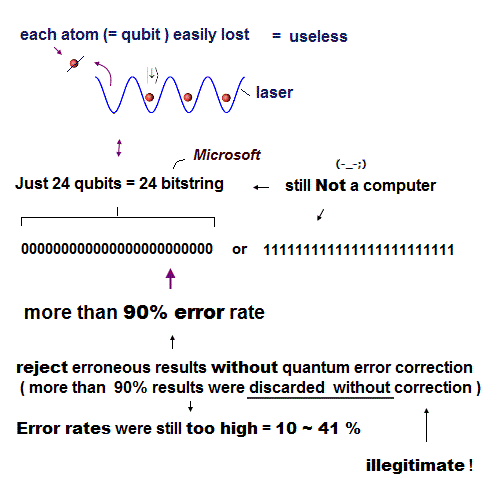As I said in Fig.19, though the sinusoidal curve of Josephson current is generated by de Broglie ( NOT electromagnetic ) effect, they neglect it and consider ONLY Coulomb relation (= I × V ).

So to know true potential curve and barrier, we have to add other effects such as de Broglie waves.
The value of Josephson energy itself needs to be reconsidered.

## Under Only electromagnetic effects, potential barrier does NOT form.

(Fig.26) Potential barrier and double-well = Effects other than Coulomb.First, if ONLY electromagnetic effects are related, double-well potential curve ( and barrier ) itself cannot form, as shown in Fig.26.
As a result, quantum tunneling itself does NOT happen.

We have to clarify the true mechanism of an integer number of magnetic flux quantum and Josephson current, to judge whether the quantum tunneling is real or not.

## Entanglement in D-Wave computer is real ?

(Fig.27) Entanglemnt among eight qubits in D-Wave ?Though it was said D-Wave quantum computer does NOT rely on quantum entanglement, the recent paper claims the entanglement effect is seen also in D-Wave machine.

But they observed ONLY eight qubits are entangled, which means "impractical".
And each qubit is just linked by coupling circuit, so ordinaly electromagnetic interations play a major role in this phenomenon.

Actually, within very short interval ( ~μm ), it is impossible to prove faster-than-light (= nonlocal ) entanglement.

## Entanglement ? is broken within 100 ns ← impractical computer.

(Fig.28) Quantum computer is very impractical.Basically, the coherence (= working ) time of flux qubit is extremely short (= about 100 nanoseconds, as shown in this site and this site (p.17) ).

Within this short time, it is impossible to use them as quantum computer and confirm their validity.
Furthermore, the temperature must be almost absolute zero (= 12.5 mK ) to work this machine.

Considering this uselessness of quantum computer ( if it really exits ) even after infinite academic papers are published, it is about time we give up quantum computer ( and entanglement ) itself and change basic ideas.

## "Cooper pair" and "phonon" are fictitious.

(Ap.1) Phonon is only a ficititous "quasiparticle".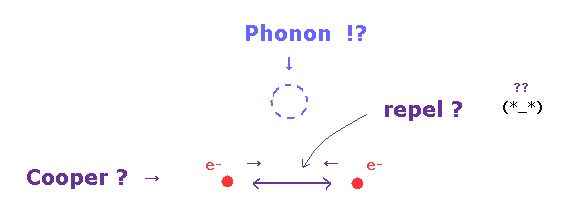The behavior of superconductor suggests that electron pairs are coupling (= Cooper pair ) over a range of hundreds of nanometers, three orders of magnitude larger than the lattice spacing, as shown on this site.

As you know, two negative electrons usually repel each other, if Coulomb law is right.
This unnatural attractive force between electrons is said to be caused by vague quasiparticle, "phonon".

But this phonon is just virtual quasiparticle, so scientists must clarify what this phonon really is, stopping "Shut up and calculate !".

## Magnetic flux quantum can be explained by quantized de Broglie wavelength.

(Ap.2) Quantized de Broglie wavelength.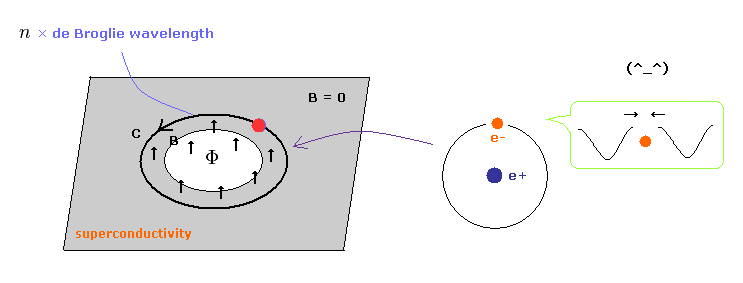Due to Meissner effect, magnetic field inside superconductor is cancelled out by electron's responding motions ( obeying Lorentz force ).
But inside a hole in a superconductor, an integer times magnetic flux quantum ( Φ = n × Φ0 ) is seen, as shown on this site.

## Quantum mechanics NEVER clarify the magnetic quantization.

(Ap.3)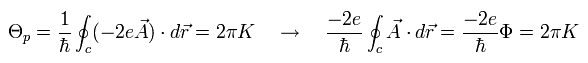As shown on this page, the current quantum mechanics tries to explain this phenomenon by quantized vector potential "A".   They insist "2e" of Ap.3 means "Cooper pair".

As shown in Ap.3, phase Θ includes de Broglie relation, so planck constant h appears, also in quantum mechanical approach.

But they never try to say what the "quantized vector potential" really means.
This troublesome attitude clearly obstructs the development of science.

## One orbit is n × de Broglie wavelength.

(Ap.4)   Lorentz force + de Broglie relation.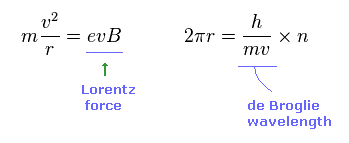If we suppose each electron is moving obeying Lorentz magnetic force (= F = evB ) and its orbital length is an integer number of de Broglie wavelength, we can describe this quantized magnetic flux naturally.

Centripetal force is equal to Lorentz magnetic force in Ap.4 left.
Ap.4 right means de Broglie relation holds in the circumference.

(Ap.5)   ← Ap.4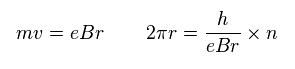Solving Ap.4, we get Ap.5.
And the total magnetic flux (= Φ ) is given by the area (= πr2 ) of this circular orbit × magnetic flux density (= B ).

So total magnetic flux (= πr2B ) is equal to an integer (= n ) number of magnetic flux quantum (= h/2e ) as shown in Ap.6.

(Ap.6) Magnetic flux quantum (= h/2e ) can be gotten from de Broglie relation.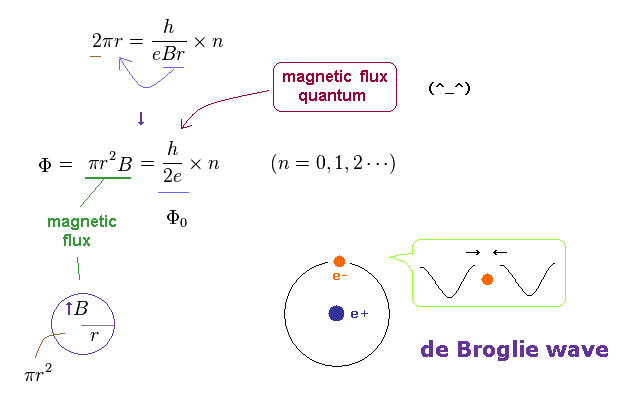As shown in Ap.6, the magnetic flux (= Φ ) through a hole is just quantized ( Φ = n × Φ0 ).
This is an origin of magnetic flux quantum without invoking Cooper pair.

So we have to think about the force (= potential ) caused by this de Broglie wave ( inteference ) to calculate the potential barrier.2014/6/23 updated. Feel free to link to this site.